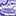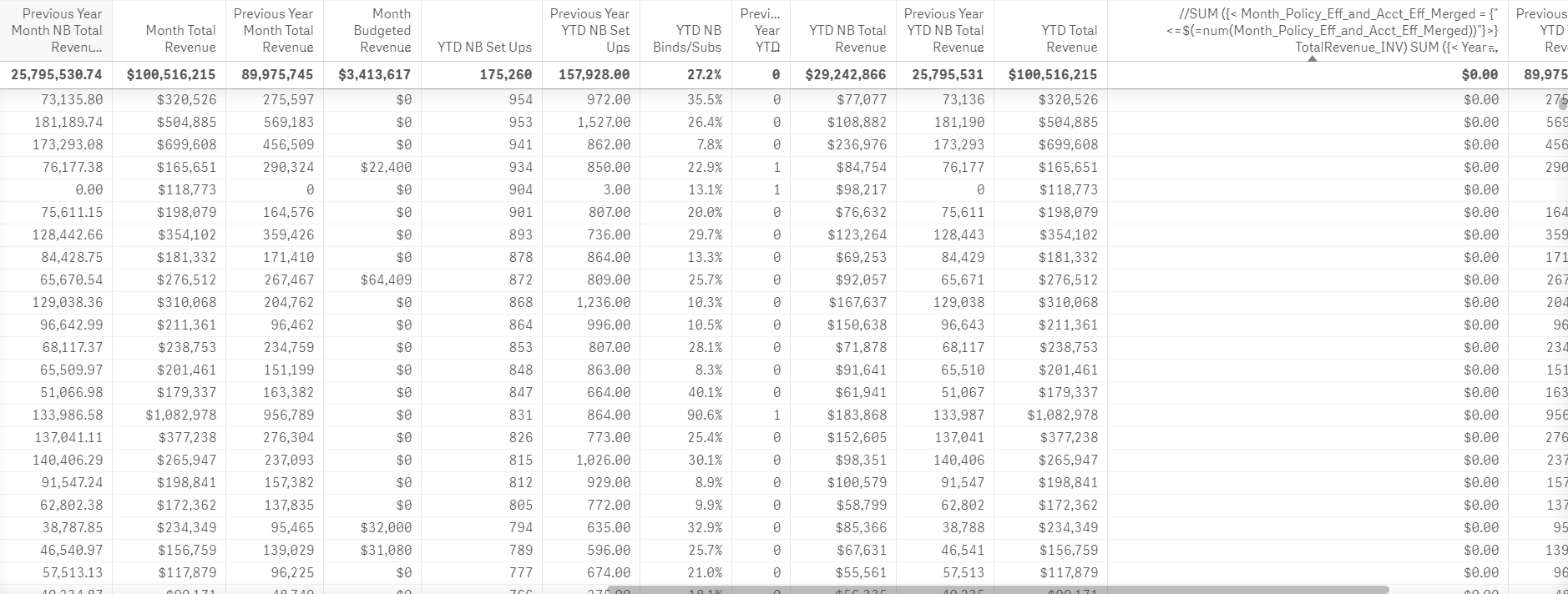# New to Qlik Sense

If you’re new to Qlik Sense, start with this Discussion Board and get up-to-speed quickly.

Announcements
Read about the latest Qlik Community enhancements on the Community News blog!
cancel
Showing results for
Did you mean:Contributor II

## YTD based on Selected Month

Hello,

I am trying to create YTD sum of revenue using a Date field (EffectiveDate) in the data set.

I tried many expressions posted in community but wasn't able to generate YTD based on month selection.

What I am looking for:

If Feb is selected then sum of revenue for Jan + Feb with a condition of BType = 'New Business'

Do I need to generate master calendar to get this done or it can be done based on a date field?

Any help would be really appreciated.

Thank you

25 RepliesContributor II
Author

I tried the above expression and all values were 0.

Expression used:

SUM ({< Month_Policy_Eff_and_Acct_Eff_Merged = {"<=\$(=num(Month_Policy_Eff_and_Acct_Eff_Merged))"} >} TotalRevenue_INV)Master II

It should work...

Do you have a sample for your app?Master II

Or try below one

SUM ({< Year=, Month=,YOURDATEFIELDHERE= {'>=\$(=YearStart(Max(YOURDATEFIELDHERE)))<=\$(=Max(YOURDATEFIELDHERE))'} >} TotalRevenue_INV)

Use your Date field in the bold text...Contributor II
Author

Didn't work.

Is there something I am doing wrong?

SUM ({< Year=, Month=,Policy_Eff_and_Acct_Eff_Merged= {'>=\$(=YearStart(Max(Policy_Eff_and_Acct_Eff_Merged)))<=\$(=Max(Policy_Eff_and_Acct_Eff_Merged))'} >} TotalRevenue_INV)

Date fields in data load editior:

Timestamp(Effective_HR,'MM/DD/YYYY') as Policy_Eff_and_Acct_Eff_Merged,

Year(Effective_HR) as Year_Policy_Eff_and_Acct_Eff_Merged,

Month(Effective_HR) as Month_Policy_Eff_and_Acct_Eff_Merged,

Screenshot of Result:Master II

First...

What are the names of your Year , Month & Quarter Fields?

You used Month_Policy_Eff_and_Acct_Eff_Merged  as your Month in previous expression

and now you used Month=,Policy_Eff_and_Acct_Eff_Merged=

Do you need a timestamp in your date field?

Maybe just use

date(Effective_HR,'MM/DD/YYYY') as Policy_Eff_and_Acct_Eff_Merged,

SUM ({< Year_Policy_Eff_and_Acct_Eff_Merged=, Month_Policy_Eff_and_Acct_Eff_Merged=, Policy_Eff_and_Acct_Eff_Merged= {'>=\$(=YearStart(Max(Policy_Eff_and_Acct_Eff_Merged)))<=\$(=Max(Policy_Eff_and_Acct_Eff_Merged))'} >} TotalRevenue_INV)

or

SUM ({< Month_Policy_Eff_and_Acct_Eff_Merged= {"<=\$(=num(Month_Policy_Eff_and_Acct_Eff_Merged))"} >} TotalRevenue_INV)Contributor II
Author

Year field Name:  Year_Policy_Eff_and_Acct_Eff_Merged

Month field name: Month_Policy_Eff_and_Acct_Eff_Merged

I don't have Quarter field.

I need timestamp for some other analysis.

I have tried both the above equations and it didn't work.

I really appreciate your help Shahbaz.Master II

Create a new Date field without stamp field

And use use that field in SUM ({< Year=, Month=,YOURDATEFIELDHERE= {'>=\$(=YearStart(Max(YOURDATEFIELDHERE)))<=\$(=Max(YOURDATEFIELDHERE))'} >} TotalRevenue_INV)

and it's ok np, a sample app would helpContributor II
Author

It is sensitive data therefore I won't be able to share sample.

I am currently in process to create Policy_Eff_and_Acct_Eff_Merged field without Timestamp and using Date field instead.

Once I have that ready, should I try this equation again as Month and Year field don't need any changes

SUM ({< Year_Policy_Eff_and_Acct_Eff_Merged=,Month_Policy_Eff_and_Acct_Eff_Merged=,Policy_Eff_and_Acct_Eff_Merged={'>=\$(=YearStart(Max(Policy_Eff_and_Acct_Eff_Merged)))<=\$(=Max(Policy_Eff_and_Acct_Eff_Merged))'} >} TotalRevenue_INV)Master II

Yes you can try, along with your newly created date field

SUM ({< Year_Policy_Eff_and_Acct_Eff_Merged=,

Month_Policy_Eff_and_Acct_Eff_Merged=,

Policy_Eff_and_Acct_Eff_Merged_Without Timestamp= {'>=\$(=YearStart(Max(Policy_Eff_and_Acct_Eff_Merged_Without  Timestamp)))<=\$(=Max(Policy_Eff_and_Acct_Eff_Merged_Without Timestamp ))'} >} TotalRevenue_INV)Contributor II
Author

Hello Shahbaz,

I tried with new date field but still I am getting zero.

Is there any alternate method to get this done?

ThanksTags
Community Browser